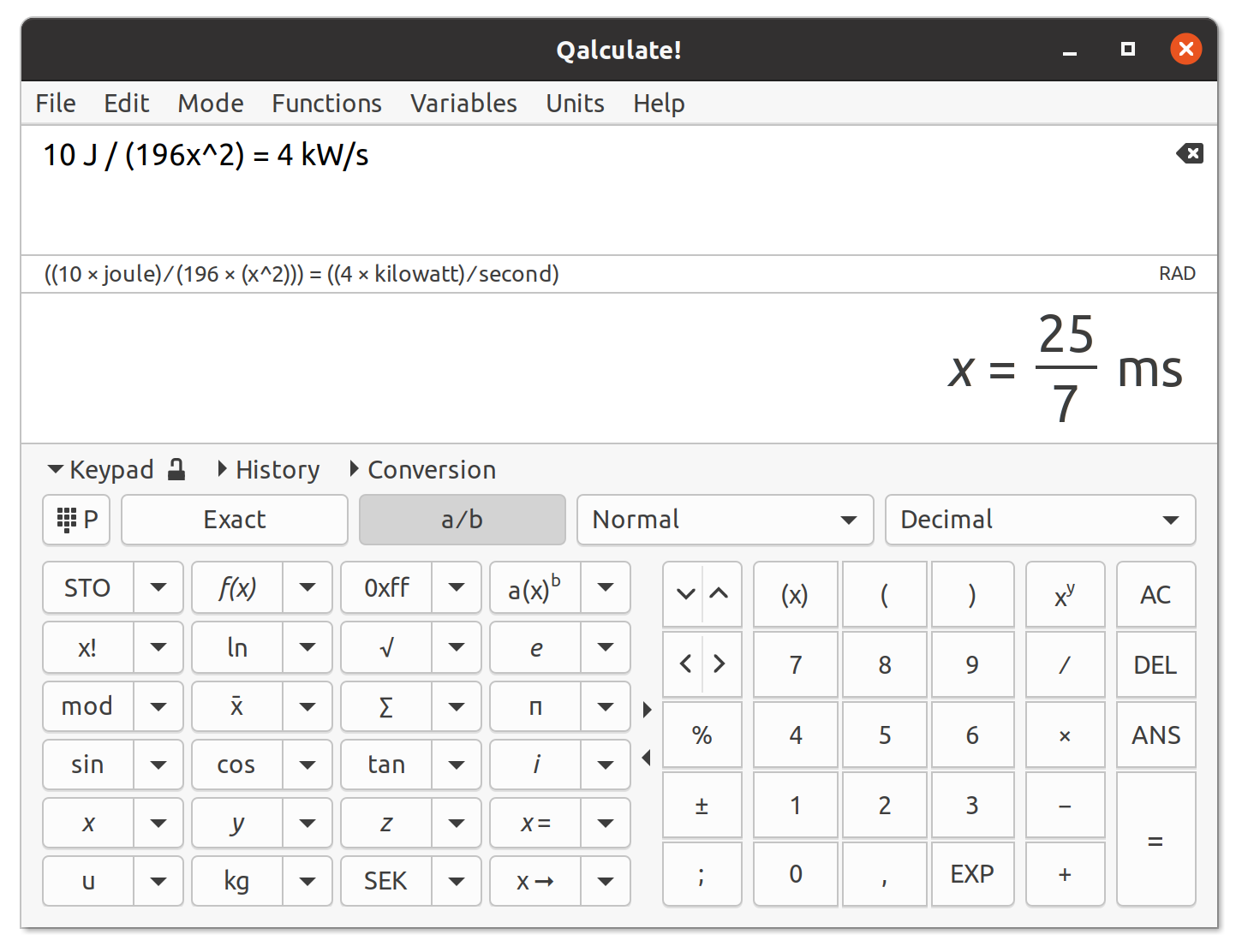# Qalculate!

## - the ultimate desktop calculatorQalculate! is a multi-purpose cross-platform desktop calculator. It is simple to use but provides power and versatility normally reserved for complicated math packages, as well as useful tools for everyday needs (such as currency conversion and percent calculation). Features include a large library of customizable functions, unit calculations and conversion, physical constants, symbolic calculations (including integrals and equations), arbitrary precision, uncertainty propagation, interval arithmetic, plotting, and a user-friendly interface (GTK+ and CLI).

Qalculate! is free software, licensed under the GNU Public License v2 (or later).

## Latest News

#### .: 2019-11-25 :. Qalculate! version 3.6 released

List of changes:
• Major improvements and fixes for integrals (note that the variable of integration argument has moved to 4th position, e.g. integrate(x^2, 1, 5) instead of integrate(x^2, x, 1, 5))
• Automatic detection of variables for undefined symbolic arguments (e.g. for diff(y^2), the derivative is calculated with respect to y instead of defaulting to x)
• Fix uncertainty propagation involving complex numbers (calculate imaginary and real uncertainty separately when using variance formula)
• Fix incorrect solutions to equations not removed
• New functions: dof() for photography depth of field calculation; awg() and awgd() for American Wire Gauge conversion; romberg() for numerical integration; randnorm(), randpoisson(), randrayleigh(), randexp(), and randuniform() for random numbers with different distributions; normdist(), expondist(), and binomdist() for distribution probability calculations
• New units: cmil and kcmil
• rand() (and related functions) now accepts an additional argument for specifying the number of random values to generate
• Option to select custom keypad or application font (GUI)
• Options to customize the window title: different modes selectable in preferences, command line option, and settitle() function (GUI)
• Minor bug fixes and feature improvements